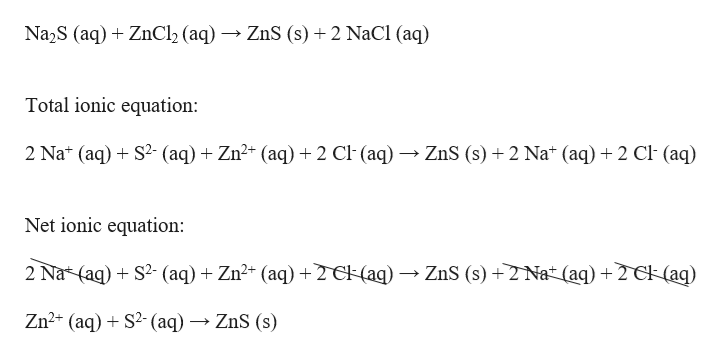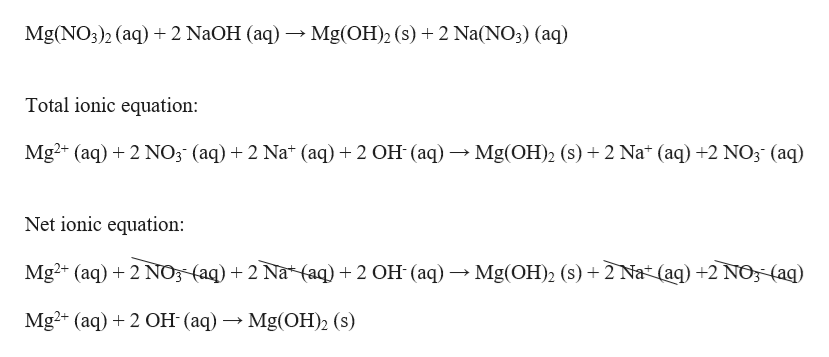Be sure to answer all parts. Write ionic and net ionic equations for the following reactions. Be sure to include the states for each species. (a) Na2S(aq) + ZnCl2(aq)   Total ionic:    Net ionic:    (b) Mg(NO3)2(aq) + 2 NaOH(aq)   Total ionic:     Net ionic:

Question
 Be sure to answer all parts. Write ionic and net ionic equations for the following reactions. Be sure to include the states for each species. (a) Na2S(aq) + ZnCl2(aq)     Total ionic:       Net ionic:       (b) Mg(NO3)2(aq) + 2 NaOH(aq)     Total ionic:         Net ionic:
Step 1

The net ionic equation shows only the chemical species that are involved in a reaction, while the complete or total ionic equation also includes spectator ions. A spectator ion is an ion that does not take part in the chemical reaction and is found in solution both before and after the reaction.

Step 2

(a) The total ionic and net ionic equation can be written as :help_outlineImage TranscriptioncloseNa2S (aq)ZnCl2 (aq) -» ZnS (s) 2 NaCl (aq Total ionic equation: 2 Na* (aq)S2 (aq)Zn2 (aq) +2 Cl (aq) -» ZnS (s)2 Na* (aq) 2 Cl (aq) Net ionic equation: 2 Na* (aq)S2(aq)Zn2 (aq) 2 C(ag) ZnS (s)2 Nat (aq) + 2 Ck(aq) Zn2 (aq)S2(aq) ZnS (s) fullscreen
Step 3

(b) The total ionic and net ionic e...help_outlineImage TranscriptioncloseMg(NO3)2 (aq +2 NaOH (aq) Mg(OH)2 (s) 2 Na(NO3) (aq) Total ionic equation: Mg2 (aq) 2 NO3 (aq) 2 Na* (aq) 2 OH (aq) Mg(OH)2 (s) 2 Na* (aq) 2 NO3 (aq) Net ionic equation: Mg2+ (aq) 2 NO (aq)2 Na* aq) 2 OH (aq) - Mg(OH)2 (s) 2 Na (aq) +2 NO, (aq) Mg2+ (aq)2 OH (aq)Mg(OH)2 (s) fullscreen

Want to see the full answer?

See Solution

Want to see this answer and more?

Our solutions are written by experts, many with advanced degrees, and available 24/7

See Solution
Tagged in

Chemistry